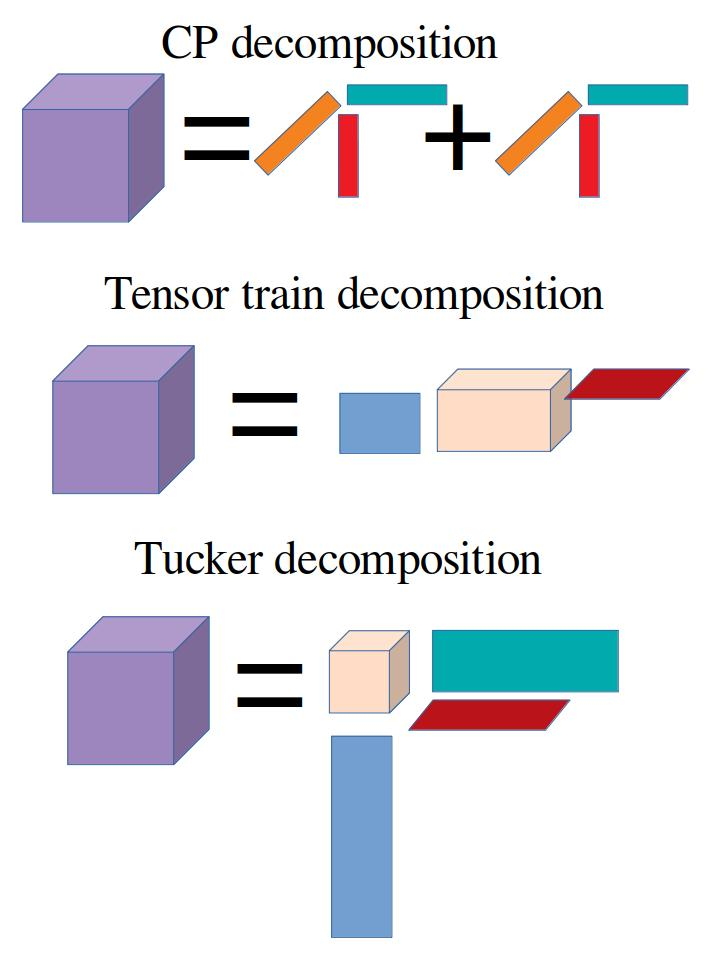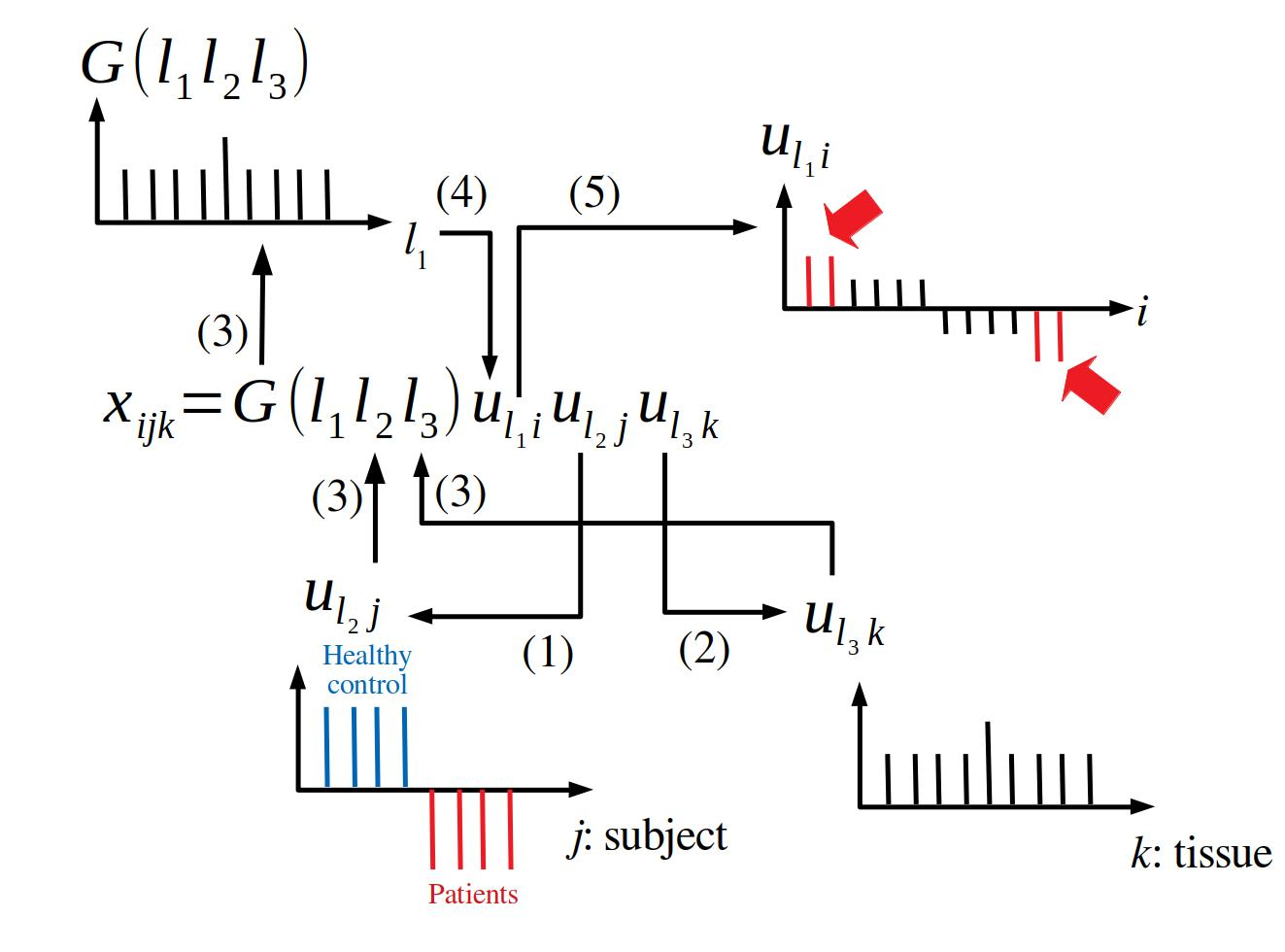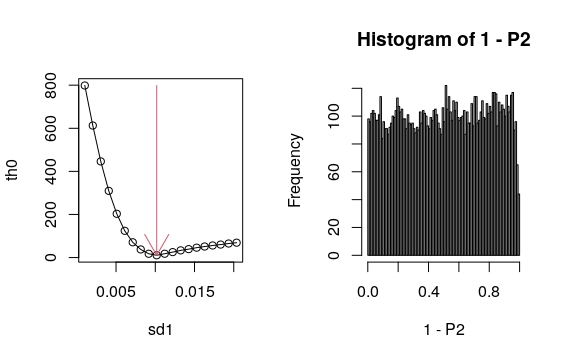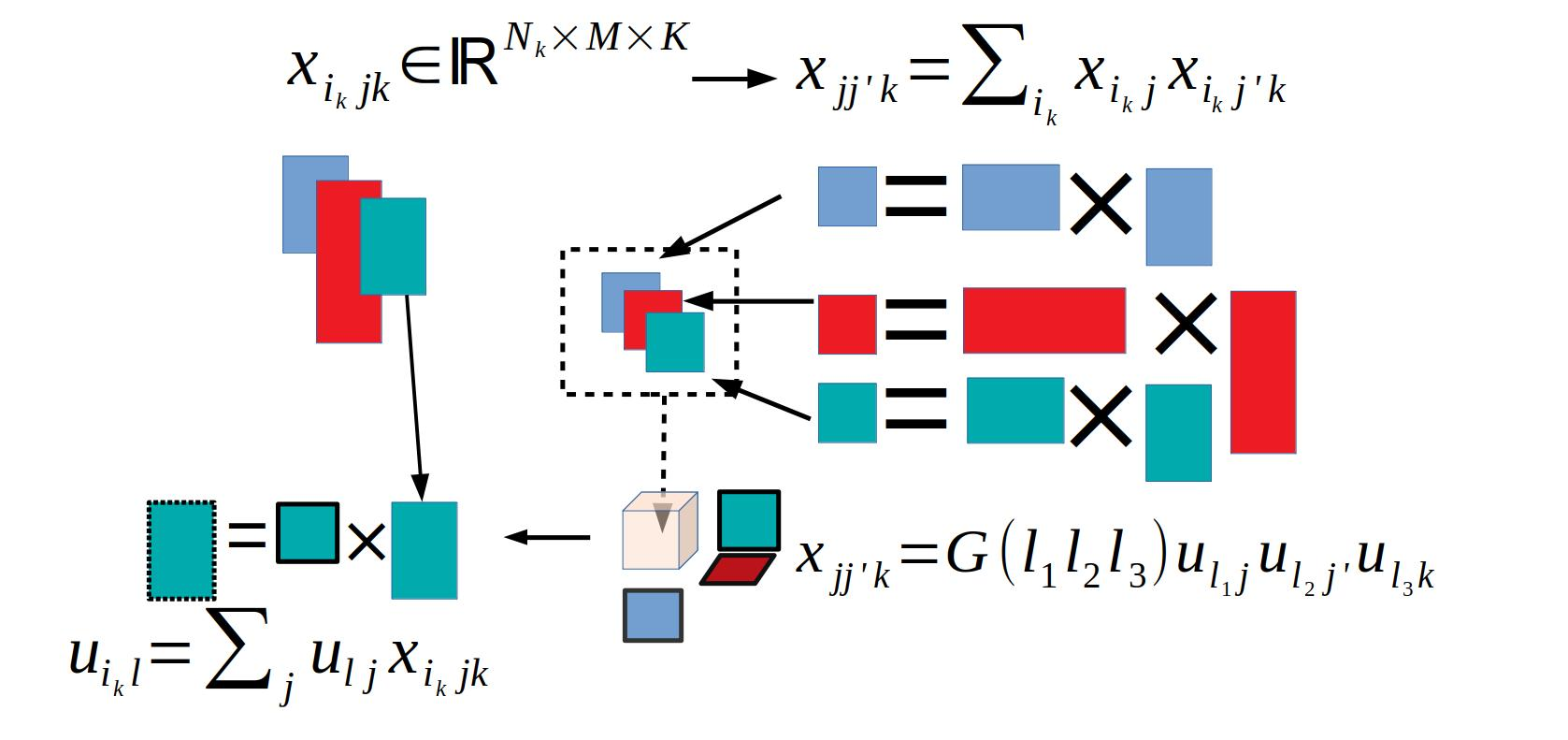# Contents

library(TDbasedUFE)

# 1 Installation

if (!require("BiocManager", quietly = TRUE))
install.packages("BiocManager")
BiocManager::install("TDbasedUFE")

# 2 Introduction

TDbasedUFE is a R package that intends to perform various analyses using Tensor decomposition based unsupervised feature extraction (TDbasedUFE) (Taguchi 2020) as much as possible in an user friendly manner. Although in the future, I am planning to implement all the features possible by TDbasedUFE in this R package, at the moment, it includes only basic features.

# 3 What are tensor and tensor decomposition?

Although [tensor] (https://bioconductor.org/packages/release/bioc/vignettes/DelayedTensor/inst/doc/DelayedTensor_1.html#12_What_is_Tensor) and [tensor decomposition] (https://bioconductor.org/packages/release/bioc/vignettes/DelayedTensor/inst/doc/DelayedTensor_1.html#13_What_is_Tensor_Decomposition) were fully described in vignettes of DelayedTensor(Tsuyuzaki 2022), we also briefly describe these.

Tensor is a natural extension of matrix that has only rows and columns, whereas tensor has more number of “rows” (or “columns”). For example, three mode tensor, $$x_{ijk} \in \mathbb{R}^{N \times M \times K}$$, has three suffix, $$i \in \mathbb{N}, j \in \mathbb{N}$$, and $$k \in \mathbb{N}$$, each of which ranges $$[1, N], [1, K]$$ and $$[1,M]$$. This representation fitted with that of genomic datasets, e. g., $$x_{ijk}$$ can represent gene expression of $$i$$th gene of $$k$$th tissue of $$j$$th subject (person). If the measurements are performed over multiple conditions, e.g., different time points, we can add additional sufix that corresponds to time. Alternatively, we can also make use of tensor to store multiomics data if we can add additional suffix that represent which of multiomics data is stored in the elements of tensor. Since the experimental design has become more and more complicated, tensor is an ideal data structure to store modern genomic observations.

Although tensor can store very complicated structure well, it does not always mean that it has ability to help us to understand these complicated structure. In order to decompose tensor, tensor decomposition was developed. There are various way to compose tensor. Usually, tensor decomposition (TD) is to decompose tensor into product of some of vectors and tensors.

The most popular TD is CP decomposition, which can decompose tensor into product some of vectors. For example, $$x_{ijk} \in \mathbb{R}^{N \times M \times K}$$ can be decomposed into the product sum of three vectors, $$\mathbb{R}^N, \mathbb{R}^M$$, and $$\mathbb{R}^K$$.Tensor Decomposition

which can be mathematically expressed as $x_{ijk} = \sum_\ell \lambda_\ell u_{\ell i} u_{\ell j} u_{\ell k}$ Alternatively, more advanced way, tensor train decomposition,
can decomposes the tensor to product of a mixture of matrix and tensor as $x_{ijk} = \sum_{\ell_1} \sum_{\ell_2} R_{\ell_1 i} R_{\ell_1 \ell_2 j} R_{\ell_2 k}$ However, in this specific study, we employed more primitive way of decomposition, Tucker decomposition, as $x_{ijk} = \sum_{\ell_1} \sum_{\ell_2} \sum_{\ell_3} G(\ell_1 \ell_2 \ell_3) u_{\ell_1 i} u_{\ell_2 j} u_{\ell_3 k}$ where $$G \in \mathbb{R}^{N \times M \times K}$$ is core tensor that evaluate the weight of product $u_{1 i} u{2 j} u{_3 k}$ and $$u_{\ell_1 i} \in \mathbb{R}^{N \times N}, u_{\ell_2 j} \in \mathbb{R}^{M \times M}, u_{\ell_3 k} \in \mathbb{R}^{K \times K}$$ are singular value matrices and are orthogonal matrices.

Although there are no uniqueness of Tucker decomposition, we specifically employed higher order singular value decomposition (HOSVD) (Taguchi 2020) to get Tucker decomposition. The reason why we employed Tucker decomposition was fully described in my book(Taguchi 2020).

## 3.1 Tensor decomposition based unsupervised feature extraction.Tensor decomposition based unsupervised feature extraction

Here we briefly describe how we can make use of HOSVD for the feature selection. Suppose $$x_{ijk}$$ represents the expression of gene $$i$$ of the $$k$$th tissue of the $$j$$th subject (person) and the task is to select genes whose expression is distinct between patients and healthy controls only at one specific tissue. In order that, we need to select $$u_{\ell_2 j}$$ attributed to $$j$$th subject which is distinct between healthy control and patients (see (1)) and $$u_{\ell_3 k}$$ attributed to $$k$$th tissue is $$k$$th tissue specific (see (2)). Then we next identify which $$G(\ell_1 \ell_2 \ell_3)$$ has the largest absolute value with fixed $$\ell_2$$ and $$\ell_3$$ that correspond to distinction between patient and healthy control and tissue specificity, respectively (see (3)). Finally, with assuming that $$u_{\ell_1 i}$$ obeys Gaussian distribution (null hypothesis), $$P$$-values are attributed to $$i$$th gene. $$P$$-values are corrected with considering multiple comparison corrections (specifically, we employed Benjamini Hochberg method(Taguchi 2020)) and genes associated with adjusted P-values less than 0.01 are usually selected (see (4) and (5)).

## 3.2 Optimization of standard deviation

Although TD based unsupervised FE was proposed five years ago, it was successfully applied to wide range of genomic science, together with the proto type method, principal component analysis (PCA) based unsupervised FE proposed ten years ago, we recently recognize that the optimization of the standard deviation (SD) in Gaussian distribution employed as the null hypothesis drastically improved the performance(Y-H Taguchi and Turki 2022) (Taguchi and Turki 2023) (Roy and Taguchi 2022). How we can optimize SD and why the optimization of SD can improve the performance is as follows. Previously, we computed SD of $$u_{\ell_1 i}$$, $$\sigma_{\ell_1}$$ as $\sigma_{\ell_1} = \sqrt{\frac{1}{N} \sum_i \left ( u_{\ell_1 i} - \langle u_{\ell_1i} \rangle\right)^2}$ $\langle u_{\ell_1 i} \rangle = \frac{1}{N} \sum_i u_{\ell_1 i}.$ However, $$\sigma_{\ell_1}$$ has tendency to be over estimated since some of $$i$$th does not follow Gaussian distribution and has too large absolute values. In order to exclude those $$i$$ that does not follow Gaussian, we tried to minimize SD of $$h_n$$ which is the frequency of $$P_i$$ computed with assuming that $$u_{\ell_1 i}$$ obeys Gaussian and falling into $$n$$th bin of histogram $$P_i$$ $\sigma_h = \sqrt{ \frac{1}{N_h} \sum_n \left ( h_n - \langle h_n \rangle \right)^2}$ where $$N_h$$ is the number of bins of the histogram of $$P_i$$ and $\langle h_n \rangle = \frac{1}{N_h} \sum_n h_n$ with excluding some of $$P_i$$ which is supposed to be too small to assume that obeys Gaussian (Null hypothesis). This is because $$h_n$$ should be constant if all of $$P_i$$ follows Gaussian (Null hypothesis).Left: $$\sigma_h$$ as a function of $$\sigma_{\ell_1}$$. Right: Histogram, $$h_n$$, of $$1-P_i$$ being computed with the optimized $$\sigma_{\ell_1}$$.

The above plot is output of examples of function selectFeature in TDbasedUFE package and represents the dependence of $$\sigma_h$$ upon $$\sigma_{\ell_1}$$ as well as the resulting histogram $$h_n$$ of $$1-P_i$$ when $$u_{\ell_1 i}$$ is fully generated from Gaussian. The smallest $$\sigma_h$$ correctly corresponds to the true $$\sigma_{\ell_1} (=0.01)$$ and $$h_n$$ is almost independent of $$n$$ as expected.

Thus it is expected that $$\sigma_h$$ will take the smallest value when we correctly exclude $$i$$s whose $$P_i$$ does not obey Gaussian (Null hypothesis). The following is the step to optimized $$\sigma_{\ell_1}$$.

1. Prepare initial $$\sigma_{\ell_1}$$ and define threshold adjusted $$P$$-value, $$P_0$$.

2. Attribute $$P_i$$s to $$i$$s with assuming Gaussian distribution of $$u_{\ell_1 i}$$ using $$\sigma_{\ell_1}$$.

3. Attribute adjusted $$P_i$$s to $$i$$s with using Benjamini-Hochberg criterion.

4. Exclude $$i$$s associated with adjusted $$P_i$$ less than $$P_0$$.

5. Compute histogram of $$1-P_i$$ and $$\sigma_h$$.

6. Modify $$\sigma_{\ell_1}$$ until $$\sigma_h$$ is minimized.

7. Finally, we identify excluded $$i$$s as the selected features because these $$i$$s do not obey Gaussian (Null hypothesis)

# 4 Multiomics data analysis

When we have multiomics data set composed of $$K$$ omics data measured on common $$M$$ samples as $x_{i_k j k} \in \mathbb{R}^{N_k \times M \times K}$ where $$N_k$$ is the number of features in $$k$$th omics data, we can make use of a tensor which is a bundle of linear kanel(Y.-h. Taguchi and Turki 2022). In this case, we can generate liner kernel of $$k$$th omics data as $x_{jj'k} = \sum_{i_k} x_{i_kjk}x_{i_kj'k} \in \mathbb{R}^{M \times M \times K}$ to which HOSVD was applied and we get $x_{jj'k} = \sum_{\ell_1} \sum_{\ell_2} \sum_{\ell_3} G(\ell_1 \ell_2 \ell_3) u_{\ell_1 j} u_{\ell_2 j'} u_{\ell_3 k}$ where $$\ell_1$$ and $$\ell_2$$ are exchangeable, since $$x_{jj'k} = x_{j'jk}$$. Singular values attributed to features can be retrieved as $u_{\ell i_k} = \sum_j u_{\ell j} x_{i_kj}$ Then the same procedure was applied to $$u_{\ell i}$$ in order to select features.Multiomics analysis

# 5 Conclusions

In this vignettes, we explain how TD based unsupervised FE can work with optimized SD. In the other vignettes, we introduce how it works well in the real examples.

sessionInfo()
#> R version 4.3.0 RC (2023-04-18 r84287)
#> Platform: x86_64-pc-linux-gnu (64-bit)
#> Running under: Ubuntu 22.04.2 LTS
#>
#> Matrix products: default
#> BLAS:   /home/biocbuild/bbs-3.18-bioc/R/lib/libRblas.so
#> LAPACK: /usr/lib/x86_64-linux-gnu/lapack/liblapack.so.3.10.0
#>
#> locale:
#>   LC_CTYPE=en_US.UTF-8       LC_NUMERIC=C
#>   LC_TIME=en_GB              LC_COLLATE=C
#>   LC_MONETARY=en_US.UTF-8    LC_MESSAGES=en_US.UTF-8
#>   LC_PAPER=en_US.UTF-8       LC_NAME=C
#>  LC_MEASUREMENT=en_US.UTF-8 LC_IDENTIFICATION=C
#>
#> time zone: America/New_York
#> tzcode source: system (glibc)
#>
#> attached base packages:
#>  stats4    stats     graphics  grDevices utils     datasets  methods
#>  base
#>
#> other attached packages:
#>   GenomeInfoDb_1.37.1  IRanges_2.35.1       S4Vectors_0.39.1
#>  BiocGenerics_0.47.0  TDbasedUFE_1.1.0     BiocStyle_2.29.0
#>
#> loaded via a namespace (and not attached):
#>   sass_0.4.6              utf8_1.2.3              bitops_1.0-7
#>   hms_1.1.3               digest_0.6.31           magrittr_2.0.3
#>   evaluate_0.21           bookdown_0.34           fastmap_1.1.1
#>  jsonlite_1.8.5          promises_1.2.0.1        BiocManager_1.30.21
#>  fansi_1.0.4             jquerylib_0.1.4         cli_3.6.1
#>  shiny_1.7.4             crayon_1.5.2            rlang_1.1.1
#>  XVector_0.41.1          bit64_4.0.5             ellipsis_0.3.2
#>  cachem_1.0.8            yaml_2.3.7              parallel_4.3.0
#>  tools_4.3.0             tzdb_0.4.0              httpuv_1.6.11
#>  GenomeInfoDbData_1.2.10 vctrs_0.6.2             R6_2.5.1
#>  mime_0.12               lifecycle_1.0.3         zlibbioc_1.47.0
#>  bit_4.0.5               vroom_1.6.3             archive_1.1.5
#>  pkgconfig_2.0.3         pillar_1.9.0            bslib_0.5.0
#>  later_1.3.1             glue_1.6.2              Rcpp_1.0.10
#>  highr_0.10              tidyselect_1.2.0        xfun_0.39
#>  tibble_3.2.1            knitr_1.43              xtable_1.8-4
#>  htmltools_0.5.5         rmarkdown_2.22          compiler_4.3.0
#>  RCurl_1.98-1.12

Roy, Sanjiban Sekhar, and Y-H. Taguchi. 2022. “Tensor Decomposition and Principal Component Analysis-Based Unsupervised Feature Extraction Outperforms State-of-the-Art Methods When Applied to Histone Modification Profiles.” bioRxiv. https://doi.org/10.1101/2022.04.29.490081.

Taguchi, Y-H. 2020. Unsupervised Feature Extraction Applied to Bioinformatics. Springer International Publishing. https://doi.org/10.1007/978-3-030-22456-1.

Taguchi, Y-H, and Turki Turki. 2022. “Adapted tensor decomposition and PCA based unsupervised feature extraction select more biologically reasonable differentially expressed genes than conventional methods.” Scientific Reports 12 (1): 17438. https://doi.org/10.1038/s41598-022-21474-z.

Taguchi, Y.-H., and Turki Turki. 2023. “Principal Component Analysis- and Tensor Decomposition-Based Unsupervised Feature Extraction to Select More Suitable Differentially Methylated Cytosines: Optimization of Standard Deviation Versus State-of-the-Art Methods.” Genomics, 110577. https://doi.org/https://doi.org/10.1016/j.ygeno.2023.110577.

Taguchi, Y-h, and Turki Turki. 2022. “Novel feature selection method via kernel tensor decomposition for improved multi-omics data analysis.” BMC Medical Genomics 15 (1): 37. https://doi.org/10.1186/s12920-022-01181-4.

Tsuyuzaki, Koki. 2022. DelayedTensor: R Package for Sparse and Out-of-Core Arithmetic and Decomposition of Tensor. https://doi.org/10.18129/B9.bioc.DelayedTensor.